## Histogram Equalization

An image histogram is a graphic representation of the frequency counts of all allowable pixel intensities. Figure 2.1 shows a sample of an image with a skewed histogram.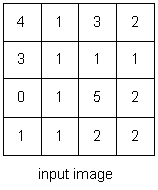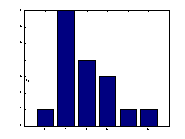Figure 2.1 A sample image with a skewed histogram (poor intensity distribution)

Since the human eye is sensitive to contrast rather than absolute pixel intensities, we would perceive less information from an image with poor intensity distributions than from the same image with better intensity distributions. Images with skewed distributions can be helped with histogram equalization (Figure 2.2). Histogram equalization is a point process that redistributes the image's intensity distributions in order to obtain a uniform histogram for the image. Histogram equalization can be done in three steps :

1. Compute the histogram of the image
2. Calculate the normalized sum of histogram
3. Transform the input image to an output image

Figure 2.2 shows the normalized sum of the image in Figure 2.1, the histogram-equalized image, and its histogram. Note that the resulting histogram is not truly uniform, but it is better distributed than before. Truly uniformed histograms for discrete images are difficult to obtained because of quantization.
 intensity sum normalized sum 0 1 1/16*5=0.31255 1 8 2.5 2 12 3.75 3 14 4.375 4 15 4.6875 5 16 5.0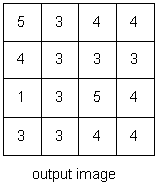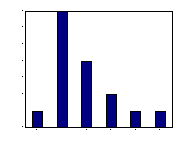Figure 2.2 A histogram-equalized sample with histogram and normalized sum

Listing 2.1 shows a MATLAB implementation of the histogram equalization function. This implementation is a table-lookup implementation.

NAME

histogram_equalize - histogram-equalizes an input image.

SYNOPSIS

histogram_equalize(input_file_name, output_file_name)

DESCRIPTION

histogram_equalize performs histogram equalization on an input image. It reads the image from the input file, computes the histogram, calculates the normalized sum, transforms the input image to an output image, and writes the output image into the output file. . The input image can be in Windows Bitmap (bmp), Hierarchical Data Format (HDF), Joint Photographic Experts Group (JPEG), Windows Paintbrush (PCX), Tagged Image File Format (TIFF), or Window Dump (XWD) format. The output file is in TIFF format.

EXAMPLES

histogram_equalize('BIRDGIRL.TIF', 'he_bg.tif')

This example (Figure 2.3) histogram-equalizes BIRDGIRL shown in Figure 1.1(a).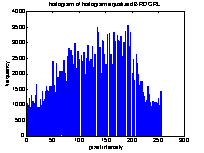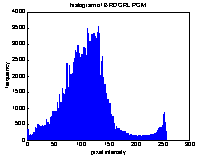a. Histogram_equalized BIRDGIRL b. Histogram of histogram_equalized BIRDGIRL c. Histogram of original BIRDGIRL

Figure 2.3 Histogram-equalized BIRDGIRL and its histogram